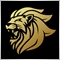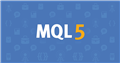# Object move with price MT516

How to move this clock close to price and make it move with price?

```//+------------------------------------------------------------------+
//|                                                        Clock.mq5 |
//|                                                                  |
//+------------------------------------------------------------------+
#property version   "1.00"
#property indicator_chart_window
#property indicator_plots 0
//+------------------------------------------------------------------+
//|                                                                  |
//+------------------------------------------------------------------+
enum VT
{
LocalTime  = 0,
ServerTime = 1
};

//--- input parameters
input VT Zone=ServerTime;   //Time
input int X=60; // Shift from the right edge
input int Y=50; // Shift from the right edge
input color TextColor=clrDodgerBlue;
MqlDateTime tt;
string Time;
int Spr;
//+------------------------------------------------------------------+
//| Custom indicator initialization function                         |
//+------------------------------------------------------------------+
int OnInit()
{
EventSetMillisecondTimer(250);
if(Zone==LocalTime) Time=TimeToString(TimeLocal(tt),TIME_SECONDS);
else Time=TimeToString(TimeCurrent(tt),TIME_SECONDS);
SetText("Clock_ind-2",Time,X,60,TextColor,16);
SetText("Clock_ind-2",Time,Y,50,TextColor,16);

return(INIT_SUCCEEDED);
}
//+------------------------------------------------------------------+
//|                                                                  |
//+------------------------------------------------------------------+
void OnDeinit(const int reason)
{
EventKillTimer();
ObjectDelete(0,"Clock_ind-2");
}
//+------------------------------------------------------------------+
//| Custom indicator iteration function                              |
//+------------------------------------------------------------------+
int OnCalculate(const int rates_total,
const int prev_calculated,
const datetime &time[],
const double &open[],
const double &high[],
const double &low[],
const double &close[],
const long &tick_volume[],
const long &volume[],
{
return(rates_total);
}
//+------------------------------------------------------------------+
//| Timer function                                                   |
//+------------------------------------------------------------------+
void OnTimer()
{
//---
if(Zone==LocalTime) Time=TimeToString(TimeLocal(tt),TIME_SECONDS);
else Time=TimeToString(TimeCurrent(tt),TIME_SECONDS);
SetText("Clock_ind-2",Time,X,60,TextColor,16);
SetText("Clock_ind-2",Time,Y,50,TextColor,16);
ChartRedraw();
}
//+------------------------------------------------------------------+
//|                                                                  |
//+------------------------------------------------------------------+
void SetText(string name,string text,int xx,int yy,color col,int r=12)
{
ObjectCreate(0,name,OBJ_LABEL,0,0,0);
ObjectSetInteger(0,name,OBJPROP_XDISTANCE,xx);
ObjectSetInteger(0,name,OBJPROP_YDISTANCE,yy);
ObjectSetString(0,name,OBJPROP_TEXT,text);
ObjectSetInteger(0,name,OBJPROP_COLOR,col);
ObjectSetInteger(0,name,OBJPROP_FONTSIZE,r);
}
//+------------------------------------------------------------------+
```Moderator
12400

Hello OBJ_LABEL uses x and y coordinates while OBJ_TEXT takes time and price values.Documentation on MQL5: Constants, Enumerations and Structures / Objects Constants / Object Types / OBJ_TEXT
• www.mql5.com
//| Creating Text object                                             |               chart_ID=0,                               sub_window=0,                              time=0,                                      price=0,                                  font_size=10,                          angle=0.0,                ...